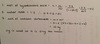Moles and Chemical Calculations Flashcards Preview

Chemisty > Moles and Chemical Calculations > Flashcards

Flashcards in Moles and Chemical Calculations Deck (108):
1

2

3

4

5

6

7

8

9

10

11

12

13

14

15

16

17

18

19

20

21

22

23

24

25

26

27

28

29

30

31

32

33

34

35

36

37

38

39

40

41

42

43

44

45

46

47

48

49

50

51

52

53

54

55

56

57

58

59

60

61

62

63

64

what is the formula of hydrated magnesium sulphate crytsals that contain MgSO4 (48.8%) and H2O (51.2%)65

When 4.76g of hydrated cobalt chloride crystals were heated they gave 2.6g of anyhydrous cobalt chloride (CoCl2). What is the formula of the hydrated salt? (Co = 59 Cl = 35.5 ; H = 1 ; O = 16)66

67

68

69

70

71

72

73

74

75

76

77

A hydrocarbon contains 62% C and the rest hydrogen. Find the empirical formula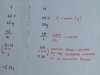78

Calculate the empirical formula for iron bromide containing 3.78g of iron and the overall ass being 20g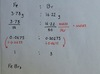79

A hydrocarbon contains 68% C and 12% H and the rest is made of oxygen. Calculate the empirical formula80

A group were given 10g of hydrated copper sulphate to heat. After heating, 6.4g of anhydrous coper sulphate remained. How many water molecules are there in hydrated copper sulphate?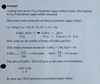81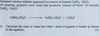82

moles of H2O is 164.1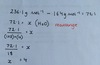8384

Dibutyl succinate is a domestic insect repellent. Its composition is 62.58% C, 9.63% H, 27.79 O. Its relative mass is 230. What are the empirical and molecular formulae of dibutyl succinate.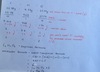85

What mass of potassium oxide is fromed when 9.75g of potassium is burned in oxygen?4K + O2 ---> 2K2O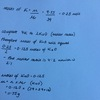86

What mass of sodium is needed to reduce 1kg of titanium chloride?Ti Cl4 + 4Na ---> Ti + 4NaCl87

What mass of silver chloride is formed when 83.25g of CaCl2 is reacted with AgNO3 solution?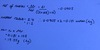88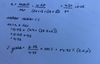89

bromeothane can be prepared by reacting hydrogen bromide with ethanol according to the followng equation:HBr + C2H5OH ---> C2H5Br +H2O( C2H5OH is ethanol)mass of ethanol is 9.20work out the percentage yield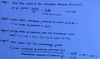90

when James reacted 5.6g of ethane with bromine water he obtained 16.9g of C2H4Br2. Calculate his % yieldC2H4 + Br2 ---> C2H4Br2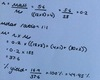91

If the percentage yield fora reaction is 100%, 60g of reactant A would make 80g of product C. How much reactant A is needed to make 80g of product C if the percentage yield of the reaction is only 75%?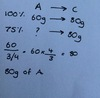9293

Calculate the atom economy of CaCO3 ---> CaO + CO294

What is a good example to use to explain the difference between % yield and atom economy?

ammonia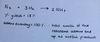95

i) What mass of copper (II) oxide will be formed if 10g of copper(II) carbonate is decomposed?CuCO3 ---> CO2 + CuOii) When 10g of copper (II) carbonate produced 4.5g of copper(II) oxide. What is the % yield?

ii) 4.5 ÷ 6.36 = 69.9%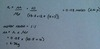96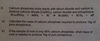b. 49.6 ÷ 4 x 10 = 12497

98

99

100

101

102

a hydrocarbon contains 18g of carbon and 3.5g hydrogen and has an RFM (Mr) of 86calculate the empirical formula of the hydrocarbon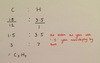103

104

which of the following contains the greatest number of hydrogen atoms?A) 2 moles of water, H2OB) 1.5 moles of ammonia, NH3C) 1 moles of hydrogen gas, H2D) 0.5 moles of methane, CH4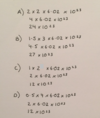105

106

which of the following gas samples occupies the greatest volume at the same temperature and pressure?Relative atomic masses: H = 1; C = 12; O = 16; F = 19; Ne = 20A) 1 gram of ethaneB) 1 gram of oxygenC) 1 gram of flourineD) 1 gram of neon

ethane, oxygen and flourine are diatomic but neon is monatomicA = 0.8 dm3B = 0.75 dm3C = 0.63 dm3D = 1.2 dm3107

17.1g of aluminium sulphate, Al2(SO4)3, was dissolved in watercalculate the number of sulfate ions, SO42-, present in the solution formed(assume the molar mass of Al2(SO4)3 is 342g mol-1 and the Avogadro Constant is 6 x 1023 mol-1)A) 3 x 1023B) 1 x 1023C) 3 x 10 22D) 9 x 10 22

D) 9 x 1022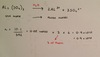108

consider the following reaction:Na2CO3 + 2HCl --> 2NaCl + H2O + CO211g of sodium carbonate is reacted with 7.3g of hydrochloric acid to produce sodium chloride, water snd carbon dioxide. Has too much sodium carbonate been used? Explain